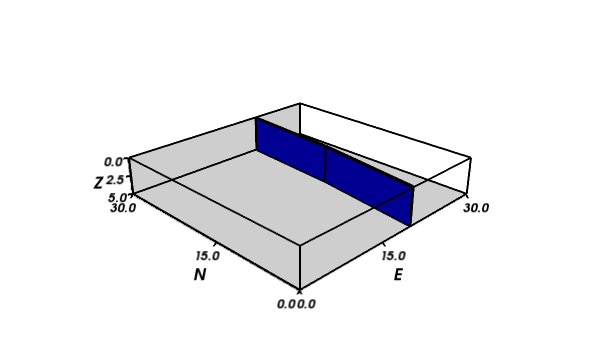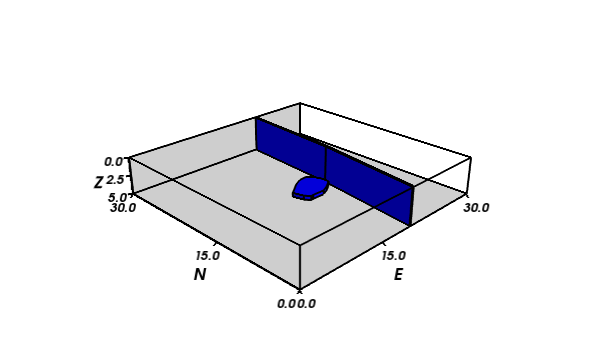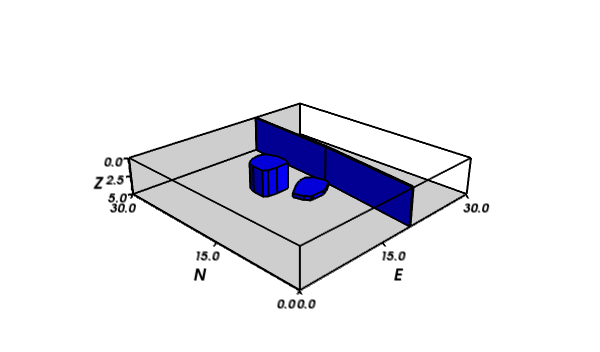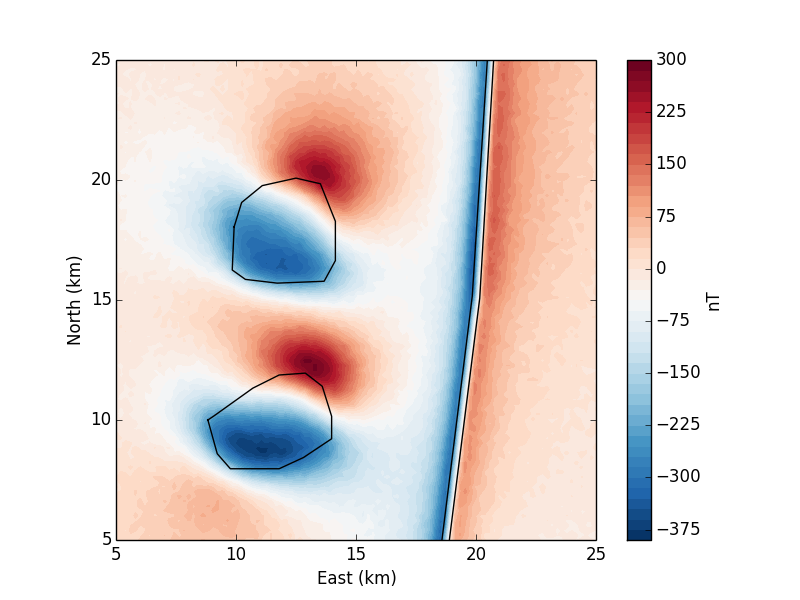# Generate synthetic magnetic data¶

This notebook generates synthetic total field magnetic anomaly data. We use the forward modeling capabilities of Fatiando a Terra to create models of polygonal prisms and calculate their total field anomaly. The data can then be saved to a file and used in other applications.

First, we must load (i.e., import) the required modules.

In :
import json
import cPickle as pickle
import numpy as np
from IPython.display import Image
from fatiando.mesher import PolygonalPrism
from fatiando.gravmag import polyprism
from fatiando  import gridder, utils
from fatiando.vis import myv, mpl
import fatiando
print("Using Fatiando a Terra version {0}".format((fatiando.version)))

Using Fatiando a Terra version 0.2


We will use function mpl.draw_polygons to interactively draw polygons on the x-y plane. These polygons will be used as the outline for the polygonal prisms. We'll draw 3 bodies in total: a dike, a sill, and an intrusive body (like a batholith).

Note that we will calculate the data inside the area specified by area but the model is contained on the larger volume bounds. We do this to minimize the unrealistic effects of the edges of the dike.

In :
area = [5000, 25000, 5000, 25000]
bounds = [0, 30000, 0, 30000, 0, 5000]


## Vertical dike¶

First, we'll draw a model of a vertical dike.

In :
mpl.figure()
mpl.axis('scaled')
mpl.square(area)
dike_verts = mpl.draw_polygon(bounds[:4], mpl.gca(), xy2ne=True)

In :
dike_verts

Out:
[[78.12500000000136, 17968.750000000004],
[15156.250000000002, 19843.750000000004],
[29843.75, 20781.250000000004],
[29843.75, 21015.625000000004],
[15078.125000000002, 20156.250000000004],
[156.25000000000136, 18281.250000000004]]

Now we use the polygon vertices drawn to create a polygonal prism model.

In :
dike = PolygonalPrism(dike_verts, 0, 5000, {'magnetization': 10})

In :
myv.figure(size=(600, 400))
myv.polyprisms([dike], linewidth=2)
myv.axes(myv.outline(bounds), ranges=[b*0.001 for b in bounds], nlabels=3, fmt='%.1f')
myv.wall_north(bounds)
myv.wall_bottom(bounds)
myv.savefig('tmp/model_dike.png')
myv.show()
Image(filename='tmp/model_dike.png')

Out:## Sill¶

Now we can draw a structure corresponding to a sill.

In :
mpl.figure()
mpl.axis('scaled')
mpl.square(area)
mpl.polygon(dike, xy2ne=True)
sill_verts = mpl.draw_polygon(bounds[:4], mpl.gca(), xy2ne=True)

In :
sill_verts

Out:
[[10000.000000000002, 8828.125000000004],
[10156.250000000002, 9062.500000000004],
[11328.125000000002, 10703.125000000004],
[11875.000000000002, 11796.875000000004],
[11953.125000000002, 12890.625000000004],
[11406.250000000002, 13593.750000000004],
[10156.250000000002, 13984.375000000004],
[9375.000000000002, 13984.375000000004],
[9218.750000000002, 13984.375000000004],
[8437.500000000002, 12812.500000000004],
[7968.750000000002, 11796.875000000004],
[7968.750000000002, 10078.125000000004],
[7968.750000000002, 9765.625000000004],
[8593.750000000002, 9218.750000000004]]
In :
sill = PolygonalPrism(sill_verts, 1000, 1500, {'magnetization': 10})

In :
myv.figure(size=(600, 400))
myv.polyprisms([dike, sill], linewidth=2)
myv.axes(myv.outline(bounds), ranges=[b*0.001 for b in bounds], nlabels=3, fmt='%.1f')
myv.wall_north(bounds)
myv.wall_bottom(bounds)
myv.savefig('tmp/model_sill.png')
myv.show()
Image(filename='tmp/model_sill.png')

Out:## Batholith¶

Finally, we draw a model of a batholith-like structure.

In :
mpl.figure()
mpl.axis('scaled')
mpl.square(area)
mpl.polygon(dike, xy2ne=True)
mpl.polygon(sill, xy2ne=True)
batholith_verts = mpl.draw_polygon(bounds[:4], mpl.gca(), xy2ne=True)

In :
batholith_verts

Out:
[[18046.875, 9921.875000000004],
[19062.5, 10234.375000000004],
[19765.625, 11093.750000000004],
[20078.125, 12500.000000000004],
[19843.75, 13515.625000000004],
[18281.25, 14140.625000000004],
[16640.625, 14140.625000000004],
[15781.250000000002, 13671.875000000004],
[15703.125000000002, 11718.750000000004],
[15859.375000000002, 10390.625000000004],
[16250.000000000002, 9843.750000000004]]
In :
batholith = PolygonalPrism(batholith_verts, 500, 4000, {'magnetization': 2})

In :
myv.figure(size=(600, 400))
myv.polyprisms([dike, sill, batholith], linewidth=2)
myv.axes(myv.outline(bounds), ranges=[b*0.001 for b in bounds], nlabels=3, fmt='%.1f')
myv.wall_north(bounds)
myv.wall_bottom(bounds)
myv.savefig('tmp/model_batholith.png')
myv.show()
Image(filename='tmp/model_batholith.png')

Out:We can group the 3 structures into a model for convenience.

In :
model = [dike, sill, batholith]


## Forward modeling¶

Now that we have a polygonal prism model, we can calculate the total field magnetic anomaly caused by the model using function polyprism.tf. Before doing that, we must specify the points where the anomaly will be calculated and the inclination and declination of the local magnetic field.

In :
shape = (100, 100)
x, y, z = gridder.regular(area, shape, z=-300)
inc, dec = -15, 30


Now we forward model the anomaly and contaminate it with pseudo-random Gaussian noise with zero mean and 5 nT standard deviation.

In :
tf = utils.contaminate(polyprism.tf(x, y, z, model, inc, dec), 5)

In :
mpl.figure()
mpl.axis('scaled')
for b in model:
mpl.polygon(b, xy2ne=True)
mpl.contourf(y, x, tf, shape, 60, cmap=mpl.cm.RdBu_r)
cb = mpl.colorbar().set_label('nT')
mpl.m2km()
mpl.xlabel('East (km)')
mpl.ylabel('North (km)')
mpl.savefig('tmp/synthetic_data.png')
mpl.show()
Image(filename='tmp/synthetic_data.png')

Out:## Output¶

Now we can save the data and model to output files.

Lets start with the data. We can use function numpy.savetxt to output an xyz file.

In :
np.savetxt('data/synthetic_data.txt', np.transpose([x, y, z, tf]))

This is what it looks like.
In :
# Can run bash commands inside the IPython notebook

5.000000000000000000e+03 5.000000000000000000e+03 -3.000000000000000000e+02 2.472022980608290510e+01
5.202020202020202305e+03 5.000000000000000000e+03 -3.000000000000000000e+02 2.136612504694526748e+01
5.404040404040404610e+03 5.000000000000000000e+03 -3.000000000000000000e+02 2.919065176934347150e+01
5.606060606060606915e+03 5.000000000000000000e+03 -3.000000000000000000e+02 2.367618454109635806e+01
5.808080808080809220e+03 5.000000000000000000e+03 -3.000000000000000000e+02 1.911091399975841654e+01
6.010101010101011525e+03 5.000000000000000000e+03 -3.000000000000000000e+02 2.005544989791811972e+01
6.212121212121213830e+03 5.000000000000000000e+03 -3.000000000000000000e+02 8.401696415669032802e+00
6.414141414141416135e+03 5.000000000000000000e+03 -3.000000000000000000e+02 2.473401968099981829e+01
6.616161616161618440e+03 5.000000000000000000e+03 -3.000000000000000000e+02 1.283030388866875526e+01
6.818181818181820745e+03 5.000000000000000000e+03 -3.000000000000000000e+02 1.549856793636272734e+01


We also need to save other information about our data (i.e., metadata), like the area, model bounds, inclination, declination, and the shape of the grid. For this, we'll use a file format called json.

In :
with open('data/metadata.json', 'w') as f:
json.dump(dict(area=area, bounds=bounds, inc=inc, dec=dec, shape=shape), f)


A json file looks like this:

In :
!cat data/metadata.json

{"shape": [100, 100], "dec": 30, "inc": -15, "bounds": [0, 30000, 0, 30000, 0, 5000], "area": [5000, 25000, 5000, 25000]}

For the polygonal prism objects (PolygonalPrisms), we need to use a different format. The Python language provides the great pickle module for object serialization. This allows us to load the same variable in another Python file from the .pickle file.

In :
with open('data/model.pickle', 'w') as f:
pickle.dump(model, f)


We'll also save the vertices that we used to create the model for later reference. We can use the json format for this.

In :
with open('data/dike.json', 'w') as f:
json.dump(dike_verts.tolist(), f)
with open('data/sill.json', 'w') as f:
json.dump(sill_verts.tolist(), f)
with open('data/batholith.json', 'w') as f:
json.dump(batholith_verts.tolist(), f)